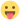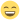## Mixed Models Module

Everything related to the development of modules in jamovi

## Re: Mixed Models Module

I'm now looking at the new version and it's looking very nice! One comment about the multinomial model coefficients table. You can group the response groups a bit nicer by using the combineBelow option for the estimates table. Basically you just do the following in your gamljgzlm.r.yaml file:
Code: Select all
`        - name: dep          title: "Response Groups"          type: text          visible: (modelSelection:multinomial)          combineBelow: true`

Another thing that can make the grouping a bit more explicit is by adding some spacing before a new response group begins by using the addFormat function.
Code: Select all
`table\$addFormat(rowKey=rowKey, col=1, Cell.BEGIN_GROUP)`

So you would only add the format when a row consists of a new response group.

You can see in this screenshot how much of a difference only adding the combineBelow statement already makes:Screenshot from 2017-11-06 18-16-39.png (166.74 KiB) Viewed 2441 timesRavi

Posts: 148
Joined: Sat Jan 28, 2017 11:18 am

## Re: Mixed Models Module

Wow, thanks a lot. I was about to say that I was ready for version 0.0.7 but your suggestions definitely call for 0.0.8. I'm going to implement the "combineBelow" which sounds a very nice option. BTW, I managed to tame nnet::multinom but I should say (WADR) it was a trip in hell. I came out of it with a new motto in R coding : honour thy parent.env() and thy parent.frame()!!!
cheers
mcmcfanda@gmail.com

Posts: 195
Joined: Thu Mar 23, 2017 9:24 pm

## Re: Mixed Models Module

Ok, done. I pushed 0.0.8 with Ravi's suggestions. Tables look better now.
mcmcfanda@gmail.com

Posts: 195
Joined: Thu Mar 23, 2017 9:24 pm

## Re: Mixed Models Module

Looks nice! I found a couple of small things1. When doing a multinomial test I get an error when doing post-hoc tests + marginal means:errorMultinom.omv
2. In mixed effects model, the contrast column of the parameter estimates table does not update when I change the factor coding. The contrast coding table does. Also, at the moment you have the default factor coding set to deviation. Is it common to use this as default (I would assume simple contrasts would be default).Screenshot from 2017-11-07 16-18-44.png (276.66 KiB) Viewed 2429 times
3. You have changed the results title of GLM to "General Linear Model", but not the options one (in the .a.yaml and .u.yaml). I'm not sure whether this is intentional, but I thought I'd mention it.Screenshot from 2017-11-07 16-22-55.png (150.77 KiB) Viewed 2429 times

But this looks really great and I think it's almost ready for a proper release. How would you like to promote it? Do you have twitter? Of course, we can help you by posting it on our twitter/facebook/linkedin/website, but I think it's good if we can retweet something you have posted so you get the attention you deserve.Ravi

Posts: 148
Joined: Sat Jan 28, 2017 11:18 am

## Re: Mixed Models Module

Hi
2-3. fixes implemented (0.0.9)
1. If I load the omv I got an error, but if I save the data in it, open the data and run it , it works fine (in my installation at least) . I probably fixed the bug producing it while updating the rest.

As regard the last point, let me get back to you after I reckon with my lack of ability to promoting thingsmcfanda@gmail.com

Posts: 195
Joined: Thu Mar 23, 2017 9:24 pm

## Re: Mixed Models Module

hi marcello,

i get the same issue as ravi, but perhaps you haven't committed all your changes just yet.

here's the stacktrace:

Code: Select all
`DebugError in X[ii, ii, drop = FALSE]: (subscript) logical subscript too longprivate\$.run()suppressWarnings({    dep <- self\$options\$dep    factors <- self\$options\$factors    covs <- self\$options\$covs    modelType <- self\$options\$modelSelection    modelTerms <- private\$.modelTerms()    if (is.null(dep) || length(modelTerms) == 0)         return()    base::options(contrasts = c("contr.sum", "contr.poly"))    data <- private\$.cleanData()    data <- mf.checkData(dep, data, modelType)    if (!is.data.frame(data))         reject(data)    for (factorName in factors) {        lvls <- base::levels(data[[factorName]])        if (length(lvls) == 1)             reject("Factor '{}' contains only a single level", factorName = factorName)        else if (length(lvls) == 0)             reject("Factor '{}' contains no data", factorName = factorName)    }    anovaTable <- self\$results\$main    estimatesTable <- self\$results\$estimates    infoTable <- self\$results\$info    formula <- jmvcore::constructFormula(dep, modelTerms)    formula <- stats::as.formula(formula)    model <- try(private\$.estimate(formula, data))    if (isError(model)) {        message <- extractErrorMessage(model)        reject(message)    }    private\$.model <- model    self\$results\$.setModel(model)    infoTable\$setRow(rowKey = "r2", list(value = mi.rsquared(model)))    infoTable\$setRow(rowKey = "aic", list(value = mf.getAIC(model)))    infoTable\$setRow(rowKey = "dev", list(value = model\$deviance))    infoTable\$setRow(rowKey = "conv", mi.converged(model))    anovaResults <- try(mf.anova(model))    if (isError(anovaResults)) {        message <- extractErrorMessage(anovaResults)        anovaTable\$setNote("anocrash", message)        STOP <- TRUE    }    rowNames <- rownames(anovaResults)    for (i in seq_along(rowNames)) {        rowName <- rowNames[i]        tableRow <- anovaResults[i, ]        colnames(tableRow) <- TCONV[["glm.f"]]        anovaTable\$setRow(rowNo = i, tableRow)    }    if (mi.aliased(model)) {        infoTable\$setRow(rowKey = "conv", list(comm = "Results may be misleading because of aliased coefficients. See Tables notes"))        anovaTable\$setNote("aliased", WARNS["ano.aliased"])        estimatesTable\$setNote("aliased", WARNS["ano.aliased"])    }    parameters <- try(mf.summary(model))    if (isError(parameters)) {        message <- extractErrorMessage(parameters)        estimatesTable\$setNote("sumcrash", message)        STOP <- T    }    ciWidth <- self\$options\$paramCIWidth/100    if (self\$options\$showParamsCI) {        citry <- try({            ci <- mf.confint(model, level = ciWidth)            colnames(ci) <- c("cilow", "cihig")            parameters <- cbind(parameters, ci)        })        if (isError(citry)) {            message <- extractErrorMessage(citry)            infoTable\$setRow(rowKey = "conv", list(value = "no"))            estimatesTable\$setNote("cicrash", paste(message, ". CI cannot be computed"))        }    }    for (i in 1:nrow(parameters)) {        estimatesTable\$setRow(rowNo = i, parameters[i, ])    }    if (STOP)         return()    private\$.populateSimple(private\$.model)    private\$.prepareDescPlots(private\$.model)    private\$.populatePostHoc(model)    private\$.populateDescriptives(model)})withCallingHandlers(expr, warning = function(w) invokeRestart("muffleWarning"))private\$.populatePostHoc(model)suppressWarnings({    none <- mf.posthoc(model, ph, "none")    bonferroni <- mf.posthoc(model, ph, "bonferroni")    holm <- mf.posthoc(model, ph, "holm")})withCallingHandlers(expr, warning = function(w) invokeRestart("muffleWarning"))mf.posthoc(model, ph, "none").posthoc.multinom(model, ph, "none")emmeans::emmeans(model, tterm, transform = "response")ref_grid(object, ...)regrid(result, transform = transform).est.se.df(object, do.se = TRUE)t(apply(object@linfct[use.elts, , drop = FALSE], 1, function(x) {    if (!any(is.na(x)) && estimability::is.estble(x, object@nbasis, tol)) {        x = x[active]        est = sum(bhat * x)        if (do.se) {            se = sqrt(.qf.non0(object@V, x))            df = object@dffun(x, object@dfargs)        }        else se = df = 0        c(est, se, df)    }    else c(NA, NA, NA)}))apply(object@linfct[use.elts, , drop = FALSE], 1, function(x) {    if (!any(is.na(x)) && estimability::is.estble(x, object@nbasis, tol)) {        x = x[active]        est = sum(bhat * x)        if (do.se) {            se = sqrt(.qf.non0(object@V, x))            df = object@dffun(x, object@dfargs)        }        else se = df = 0        c(est, se, df)    }    else c(NA, NA, NA)})FUN(newX[, i], ...).qf.non0(object@V, x)`jonathon

Posts: 1277
Joined: Fri Jan 27, 2017 10:04 am

## Re: Mixed Models Module

Hi Marcello,
I'm looking at the new version (gamlj 0.0.9), if I first use your form, post-hoc error for missing the lsmeans package.

If I do other modules that use it first (post-hoc ANOVA), it also works with your module.
Also reading previous msgs, I've copied lsmeans into gamlj/build/R and recompiled.
Fixed error.

Thanks a lot, you are doing a great job.
Cheers, Maurizio.
AttachmentsScreenshot 2017-11-07 23.21.52.png (114.7 KiB) Viewed 2409 timesMAgojam

Posts: 74
Joined: Thu Jun 08, 2017 2:33 pm
Location: Parma (Italy)

## Re: Mixed Models Module

hey marcello, couple of other things

typos:

generalized linear models => model info => identity not indentity
generalized linear models => 'analysis of deviance' not 'analisys of deviance'

i'd recommend avoiding verbs in the names/titles of options:

i.e. "Contrasts in estimates table", rather than "Show contrasts ..."
"Confidence intervals" rather than "Show CI", etc.

"Show" is implied by the checkbox.

cheersjonathon

Posts: 1277
Joined: Fri Jan 27, 2017 10:04 am

## Re: Mixed Models Module

hey,
@jonathon : fixed and (hopefully) committed and pushed

@maurizio: Yes, you're right. I've moved from lsmeans:: to emmeans: (the new version of the lsmeans package) but I did not clean up all the references. Now it should be ok.mcfanda@gmail.com

Posts: 195
Joined: Thu Mar 23, 2017 9:24 pm

## Re: Mixed Models Module

looking good! let me know when you want us to push a new version to the library.

you could bump the version number up a bit too if you wanted. something to signal to people that this is more than an alpha.

jonathonjonathon

Posts: 1277
Joined: Fri Jan 27, 2017 10:04 am

PreviousNext